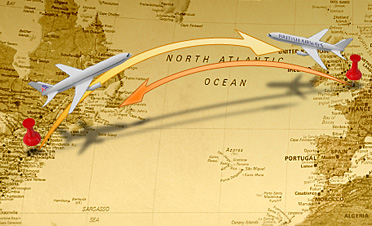Turning PointA plane traveling from point A to point B with a tailwind decides to turn around at point P. P is defined as that point where the return trip to A would take the same time as the trip from point P to point B. Enter Total distance, Wind speed and Plane speed and click Submit. Total distance : Wind speed : Plane speed : Distance to point P is : Extra credit Say the trip from A to B takes 3 2/3 hours and the trip from B to A takes 5 hours. If the wind speed is 40 miles per hour, what would be the distance from point A to the point P as defined above? Extra Extra credit Either by looking at the source code or by using Algebra, what is the equation that shows what d is as a function of t, w and p where d is the distance from point A to point P, t is the total distance between point A and point B, w is the wind speed and p is the plane speed? Please email your answer to the Extra credit problem(s) to bradley_lee_r@sbcglobal.net. If you would like to see my solutions, go to Solution.